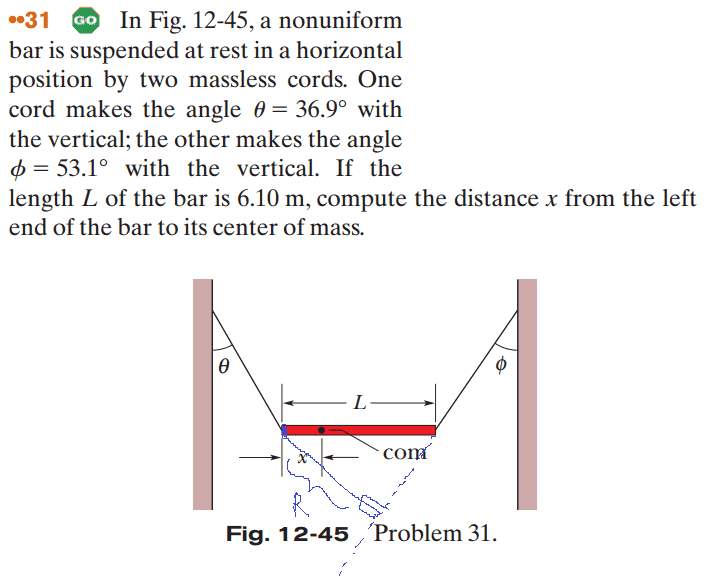How to find the 'r' for Torque

Homework StatementHomework Equations

Torque = r * F * sin(x)

The Attempt at a Solution

The solution says that the 'R' I marked was L*cos(phi). I fail to see the geometry of how they got this...you have only one side and one right angle for that triangle...how can you solve for 'R'?